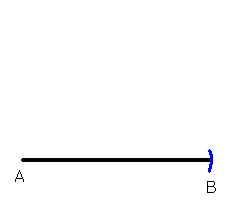# Hammond Maths- Ruler and compass constructions (revision world)

?

## Ruler and Compass Constructions

You may need to know how to perform various constructions using a pair of compasses and an unmarked ruler (a ‘straight-edge’). When doing this sort of thing, you are not allowed to use any measuring equipment! You have to use the compasses to do the measuring...

You usually start by drawing a straight line with your ruler, or you might be given a straight line in an exam to start with.

Constructing an Equilateral Triangle

Suppose you have been given a line, AB, and you have to construct an equilateral triangle which has AB as one of it’s sides (an equilateral triangle is a triangle whose sides are all the same length).

Step 1: Put the compass point on A and open it so that the pencil touches B. So you have "measured" the length of AB with the compasses. Be careful not to open or close the compasses at all now!Step 2: Leaving the compass point on A, draw an arc with the pencil roughly where you think the other vertex (corner) of the triangle is going to be. The distance from A to this arc is going to be the same as the length of AB. Therefore since we…